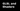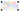Navigate back to the homepage

# Real Time Volumetric Clouds, GLSL and Three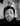Rick
March 27th, 2021 · 5 min read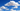This is an experiment I did at the start of 2021 in the depths of winter . I thought to my self, I know.. how can I occupy my time 🤔 … spend my evenings making clouds :)

I did alot of reading around the topic, studied alot of GLSL and read alot of white papers on noise and clouds, sad I know haha. And found SHADERed! A god send for debugging, I would not have been able to do this without SHADERed!

A little disclaimer, if things aren’t 100% accurate, well this is because I’m still learning and studying glsl. So apologies in advance if anything isn’t quite right.

Anyhow, the aim here is to use post-processing and try to get something which resembles clouds. Here is an example of what we are aiming for

As you can see this was quite a successful experiment in modelling clouds!

This is literally an experiment… I know for starters it would be a very good idea to use tiled 3D noise, buttt the point of this is an experiment and hopefully one way to approach clouds 🙏

Here’s the high level overview of what we are going to go over:

• Three setup (we aren’t positioning over a 3D scene, it will all be fixed for now)
• Post-processing
• The vertex and Fragment shaders

## Three setup

Here is the repo, so if you want to take a quick look, go take a tour. The repo uses react three fiber (R3F) and the effect composer. With this setup I have used create react app (CRA) and bootstrapped it with R3F. It is a super simple setup as we are only interested in exploring post-processing and clouds!

Here is the folder structure: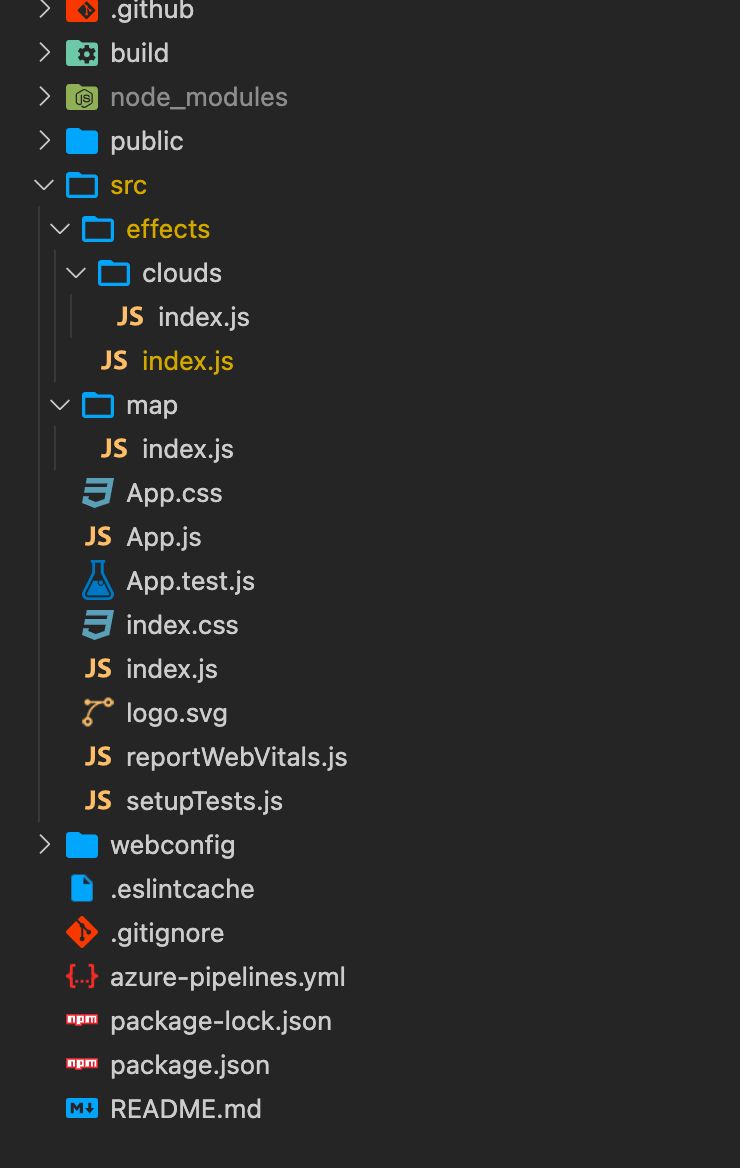Here is the Effect Composer:

```.css-1chxjt6{position:absolute;right:22px;top:24px;padding:8px 12px 7px;border-radius:5px;color:#6f7177;-webkit-transition:background 0.3s ease;transition:background 0.3s ease;}.css-1chxjt6:hover{background:rgba(255,255,255,0.07);}.css-1chxjt6[data-a11y="true"]:focus::after{content:"";position:absolute;left:-2%;top:-2%;width:104%;height:104%;border:2px solid var(--theme-ui-colors-accent,#6166DC);border-radius:5px;background:rgba(255,255,255,0.01);}@media (max-width:45.9375em){.css-1chxjt6{display:none;}}1import { SavePass } from 'three/examples/jsm/postprocessing/SavePass';2import { RenderPass } from 'three/examples/jsm/postprocessing/RenderPass';3import * as THREE from 'three';4
5import { clouds } from './clouds';6
7extend({ EffectComposer, ShaderPass, SavePass, RenderPass })8
9const Effects = () => {10
11  const composer = useRef();12  const cloudsPass = useRef()13  const { scene, gl, size, camera, clock } = useThree()14
15  useEffect(() => composer.current.setSize(size.width, size.height), [size])16
17  useFrame(() => {18    if (cloudsPass.current) {19        cloudsPass.current.uniforms['uResolution'].value = new THREE.Vector2(20            size.width,21            size.height22        );23      cloudsPass.current.uniforms['uTime'].value = clock.elapsedTime;24    }25    composer.current.render()26  }, 1);27
28  return (29    <effectComposer ref={composer} args={[gl]}>30        <renderPass31            attachArray="passes"32            scene={scene}33            camera={camera}34        />35        <shaderPass36            attachArray="passes"37            ref={cloudsPass}38            args={[clouds]}39            needsSwap={false}40            renderToScreen41        />42    </effectComposer>43  )44}45
46export default Effects;```

### Effect Composer

A quick tour of the effect composer is in order.. Firstly we wrap it all in an effects component, a standard react component. We then define a render pass which renders the scene. After which we define a shader pass where all the magic happens.

The premise behind this is we create a 3D mathematical version of our environment. What does this mean?

When we talk about 3D, everything is maths. We model how the environment looks using maths. This isn’t as scary as it sounds.. Here are the components of our fragment shader:

• ray marching algorithm
• SDF function
• density function
• And most importantly noise and fbm!

Very quickly the explanation is this.. for every pixel we fire a ray out into 3D space, when this ray hits an object (defined by our SDF function) we start to calculate colours, densities and lighting.

These positions where we hit a point in 3D space within our spherical shapes, defines the shape of our ray-marched object, remember this happens for every pixel.

After this we can use a density and lighting function, this will blend the cloud colour with the background colour (in our case) or… do a texture lookup of our scene texture and blend it in with that.

Why would you do this? So imagine we have a camera looking down into a scene through clouds, you wouldn’t want to use an arbitrary colour you would want to use the colour of our scene.

How do we get clouds? well… layering noise. If you do some googling you might hear the word octaves. An octave of noise is a layer of noise (this is in layman’s terms so don’t go ape on me), a 2D look of layed noise is here:Looks cloudy ey 😃 well imagine this in 3D space!

So first things first here is the fragment shader..

```1uniform vec2 uResolution;2uniform float uTime;3
4vec3 sundir = vec3(4.0,10.0,4.0);5const int OCTAVES = 2;6vec3 backgroundColor = vec3(1.0);7
8// Noise Related Functions9float mod289(float x){return x - floor(x * (1.0 / 289.0)) * 289.0;}10vec4 mod289(vec4 x){return x - floor(x * (1.0 / 289.0)) * 289.0;}11vec4 perm(vec4 x){return mod289(((x * 34.0) + 1.0) * x);}12float noise(vec3 p){13    vec3 a = floor(p);14    vec3 d = p - a;15    d = d * d * (3.0 - 2.0 * d);16    vec4 b = a.xxyy + vec4(0.0, 1.0, 0.0, 1.0);17    vec4 k1 = perm(b.xyxy);18    vec4 k2 = perm(k1.xyxy + b.zzww);19    vec4 c = k2 + a.zzzz;20    vec4 k3 = perm(c);21    vec4 k4 = perm(c + 1.0);22    vec4 o1 = fract(k3 * (1.0 / 41.0));23    vec4 o2 = fract(k4 * (1.0 / 41.0));24    vec4 o3 = o2 * d.z + o1 * (1.0 - d.z);25    vec2 o4 = o3.yw * d.x + o3.xz * (1.0 - d.x);26    return o4.y * d.y + o4.x * (1.0 - d.y);27}28float PHI = 1.61803398874989484820459;  // Φ = Golden Ratio29float gold_noise(in vec2 xy, in float seed){30    return fract(tan(distance(xy*PHI, xy)*seed)*xy.x);31}32float random( vec3 scale, float seed ){33return fract(34        sin(35            dot( gl_FragCoord.xyz + seed, scale )36        ) * 43758.5453 + seed37    ) ;38}39
40// Fractal Brownian Noise41float fbm(vec3 x, int octaves) {42    float v = 0.0;43    float a = 0.5;44    vec3 shift = vec3(100);45    for (int i = 0; i < octaves; ++i) {46        v += a * noise(x);47        x = x * 2.0 + shift;48        a *= 0.5;49    }50    return v;51}52// SDF function53float sphereSDF (vec3 position, float radius) {54    vec3 origin = vec3(.0) ;55    return length(origin - fbm(position, 1)  ) - radius;56}57
58// MiN/Max for inside bounding box59float value = 2.0;60float xMin = -2.0;61float xMax = 2.0;62float yMin = -2.0 ;63float yMax = 2.0;64
65bool insideCuboid (vec3 position) {66    float x = position.x;67    float y = position.y;68    return x > xMin && x < xMax && y > yMin && y < yMax;69}70
71float densityFunc(const vec3 p) {72    vec3 q = p;73    // Move the noise by adding vector and multiplying by74    // increasing time.75    q += vec3(0.0, 0.0, -.20) * uTime;76    float f = fbm(q, OCTAVES);77    return clamp( f - 0.5  , 0.0, 1.0 );78}79vec3 lighting(const vec3 pos, const float cloudDensity80, const vec3 backgroundColor, const float pathLength ) {81    // How light is it for this particular position82    float clampedDensity = clamp(cloudDensity, 0.0, 1.0);83
84    // How light is it for this particular position85    vec3 lightnessFactor = vec3(0.91, 0.98, 1.0) +86        vec3(1.0, 0.6, 0.3) * 2.0 * clampedDensity;87
88    // Calculate a darkness factor based on density89    vec3 darknessFactor = mix(90        vec3(1.0, 0.95, 1.0),91        vec3(0.0, 0.0, 0.00),92        cloudDensity93    );94
95    const float transmittanceFactor = 0.0003;96
97    // As pathLength increases ie you get further away98    // from the origin of thr ray firing into 3D space99    // the transmittance will decreasse. Meaning the100    // color wont be as bright. Took alot of fiddling around..101    float transmittance = exp( -transmittanceFactor * pathLength );102
103    // Here we mix the background color with the combined104    // lightness and darkness, based on transmittance.105    return mix(106        backgroundColor,107        darknessFactor * lightnessFactor,108        transmittance109    );110}111void main() {112    // Taking into account the screen resolution113    vec2 uv = (gl_FragCoord.xy-.5*uResolution.xy)/uResolution.y;114
115    // Ray Marching Variables116    vec3 rayOrigin = vec3(uv, 1.0);117    vec3 rayDirection = vec3(uv, 1.0);118    vec4 colorSum = vec4(0.0);119    float rayDistanceToSphericalShape = 0.0;120    float MAX_DISTANCE = 100.0;121
122    // Use loop to increase distance of ray123    // on each iteration.124    for (int i = 0; i< 120;i ++) {125        // Get currentStep or tip of ray126        vec3 currentStep =127            rayOrigin +128            rayDirection *129            rayDistanceToSphericalShape;130
131        // Mimic movement of cloud like shapes by132        // moving the step in a certain direction133        // which increases as time increases134        vec3 movedStepWithTime =135            currentStep +136            vec3(0.0,0.0,-0.6) * uTime;137
138        // Calculate if tip of ray is within one of our139        // noise affected 3D special shapes, defined by the SDF140        // function.141        float dist = sphereSDF(movedStepWithTime, .95);142
143
144        // Limit the clouds to a certain box, only within145        // this box will be rendered.146        bool insideBoundries = insideCuboid(movedStepWithTime);147
148
149        // The color sum is basically where each time the ray gets150        // increased in distance and the tip of the ray is inside151        // spherical cloud shape (defined by our SDF), we add the152        // colors up. I tried to make it so that the middle of a153        // cloud would be a more solid color and the outskirts154        // would have a less bright color. If opacity is > 0.99155        // we dont want to run the code anymore it has reached156        // max opaquness.157        if( 0.99 < colorSum.a ) break;158
159        // Calculate The density of this rays position160        float cloudDensity = densityFunc( currentStep );161
162        if (dist < 0.1002 && insideBoundries ) {163            // Get color of tip of ray within spherical shape164            vec3 colorRGB = lighting(165                movedStepWithTime,166                cloudDensity,167                backgroundColor,168                dist169            );170
171            // tweak opacity or alpha172            float alpha = cloudDensity * 0.9;173
174            // Multiply color by alpha175            vec4 color = vec4(colorRGB * alpha, alpha);176
177            // Add color to colorSum allowing us to178            // account for layers of clouds.179            colorSum += color;180
181
182        }183
184        if (rayDistanceToSphericalShape > MAX_DISTANCE) {185            break;186        }187
188        // When we fire the ray, if the distance to out sphercial189        // shapes is less than 0.0001 then we add the distance to the190        // overall rayDistanceToSphericalShape and bypass the need191        // to in crease by 0.1 each time. This way we speed things up.192
193        rayDistanceToSphericalShape += dist < 0.0001 ? dist : 0.1;194
195    }196
197    vec3 backgroundSky = vec3( 0.7, 0.79, 0.83);198    vec4 finalColorSum = colorSum;199
200    // Blending the background color with the colorSum201    vec3 finalColor =202        backgroundSky * ( 1.0 - finalColorSum.a ) + finalColorSum.rgb;203    gl_FragColor = vec4(finalColor, 1.0);204
205}```

### Noise

Don’t run away as it is about to get interesting with some explanations of the madness 😎 I am not going into an in depth explanation more like a overview and I have commented the fragment shader, which should hopefully give you an idea of whats going on!

So we have some uniforms and a 3D noise function, the function for 3D noise was found online here.

When we provide this 3D noise function with a vec4 we get out a random noise value. We use this in two ways, one for the shape of our sphere and also for the colour of the cloud. The first thing which needs explaining is ray marching.

## Ray Marching

The very essence of this is firing a ray from an origin and when it hits a specific part of the 3D space we make note of the position at which the ray is inside the spherical shape. Here is a basic raymarching algorithm:

```1vec2 uv = (gl_FragCoord.xy-.5*uResolution.xy)/uResolution.y;2// .....3vec3 rayOrigin = vec3(uv, 1.0);4vec3 rayDirection = vec3(uv, 1.0);5
6float rayDistanceToSphericalShape = 0.0;7float MAX_DISTANCE = 100.0;8
9for (int i = 0; i< 120;i ++) {10    // Get currentStep or tip of ray11    vec3 currentStep = rayOrigin + rayDirection * rayDistanceToSphericalShape;12
13     float currentStepToSphere = sphereSDF(currentStep, .95);14
15    if (currentStepToSphere < 0.1) {16        // do Something inside the spherical shape17    }18
19    if (rayDistanceToSphericalShape > MAX_DISTANCE) {20        break;21    }22
23    rayDistanceToSphericalShape += currentStepToSphere < 0.0001 ? currentStepToSphere : 0.1;24
25}26
27// ......```

We have the origin, ray direction and a loop. One of the important notes here is we use gl_FragCoord to create the direction and origin. gl_FragCoord is a built in value added by three.

This is good because the gl_FragCoord has a coordinate for every pixel on the screen. So in essence we fire a ray into the scene from every pixel on the screen. With this we have a nice setup to ray-march.

We add the origin to the direction to point in the right direction and either the max distance to the spherical shape or an arbitrary step value:

```1vec3 currentStep = rayOrigin + rayDirection * rayDistanceToSphericalShape;2
3//.......4
5rayDistanceToSphericalShape += currentStepToSphere < 0.0001 ? currentStepToSphere : 0.1;```

So what is the currentStepToSphere? We find out the distance to our spherical shapes:

```1float sphereSDF (vec3 position, float radius) {2    vec3 origin = vec3(.0) ;3    return length(origin - fbm(position, 1)  ) - radius;4}5//.......6
7// Calculate if tip of ray is within one of our8// noise affected 3D special shapes, defined by the SDF9// function.10float currentStepToSphere = sphereSDF(movedStepWithTime, .95);```

If this value is negative and we are in the spherical shapes.. we start to figure out colours, densities and alpha’ness or opacity 👌 Lets take a deeper dive into SDF’s.

## Shape of Spherical shapes (or clouds)

Here is a couple of great places for SDF functions: here and here.

Lets take a sphere as an example: We need some basic vector maths…

```1// if we want to find out vector A to B 2B - A = AB 3
4length(AB) = distance;5
6// Therefore position to origin:7origin - Position 8
9// get a distance or magnitude 10length(origin -Position)```

and here’s the magical part, we minus the radius of our sphere..

```1length(origin - position) - radius;2
3// e.g.4// if radius is 9.0:5length(vec3(0.0, 0.0, 0.0) - vec3(0.0, 0.0, 11.0)) = 116
711 - 9 = 3;8
9// Therefore oustide sphere!10
11// if radius is 12.012length(vec3(0.0, 0.0, 0.0) - vec3(0.0, 0.0, 11.0)) = 11.013
1411.0 - 12.0 = -1.0;15
16// Therefore inside sphere!```

If the resulting float is negative then we are inside the sphere, if it is positive then we are still outside of the sphere and we can carry on ray-marching!

You may have noticed we apply noise to the origin:

`1return length(origin - fbm(position, 1)  ) - radius;`

The fbm is layering one layer of noise ontop of another as mentioned before. This basically morphs the shape of our sphere and places many origins across our scene, for every position we input we apply noise and this essentially means the origin moves from one vec3 to another, giving the appearance of random spherical 3D shapes… almost a match made in heaven if we are trying to get cloudy shapes!

Here is an example video showing some prelim attempts with Applying noise to an SDF function:

## How to determine the color

First off we need the density of the cloud. We generate some noise and clamp this between 0.0–1.0. 0.0 means darker and 1.0 means lighter (0.0 = black, 1.0= white).

Then in the lighting function we end up with this:

`1return mix(backgroundColor, darknessFactor * lightnessFactor, transmittance );`

This took a lot of playing around but we linearly interpolate the background colour with the overall colour of the cloud. The factor which determines how much we mix is transmittance.

The transmittanceFactor is a very small number, 0.0003.

Lets run through some examples of transmittance:

```1exp( -transmittanceFactor * pathLength );2
3exp( -0.0003 * 1.0 ) = 0.99970004499;4
5exp( -0.0003 * 50.0) = 0.9851119396;6
7exp( -0.0003 * 100.0 ) = 0.97044553354;```

This mimics that the closer certain types of cloud get to the human eye the less blocky the colour is.

The final act once we have the colorSum, after all our loop iterations.. is adding the background colour with the sumColor, this is how we give the appearance of clouds blending into the background colour:

```1vec3 backgroundSky = vec3( 0.7, 0.79, 0.83);2vec4 finalColorSum = colorSum;3
4// Blending the background color with the colorSum5vec3 finalColor = backgroundSky * ( 1.0 - finalColorSum.a ) + finalColorSum.rgb;6gl_FragColor = vec4(finalColor, 1.0);```

## Final Thoughts

This was an experiment on how one could go about doing volumetric clouds.

Here is the video of the less intensive clouds:

I suppose they aren’t amazing, but we cannot increase the octaves of noise on a less powerful computer. If you have a unit of a computer feel free to clone the repo and mess around with the octaves and settings. I’m sure you will be able to tweak it 😎.

We have gone through loads of stuff here:

• noise, octaves, layering and fractal brownian motion
• ray marching
• examples of more complicated glsl syntax
• and of course show casing some volumetric clouds

If you have any questions feel free to contact me. I did this in January and spent alot of late nights playing around with code and SHADERed! is an excellent tool which actually allows you to debug shader code :))

So I might not have all the answers 👀 but I can sure try and help explain things more.

I hope you enjoyed the whistle stop tour of Volumetric Clouds and GLSL..

Until next time, stay safe!

### More articles from theFrontDev